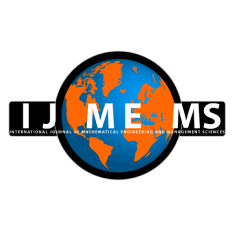### International Journal of Mathematical, Engineering and Management Sciences

#### ISSN: 2455-7749

New Oscillation Conditions for Second Order Half-Linear Advanced Difference Equations

#### New Oscillation Conditions for Second Order Half-Linear Advanced Difference Equations

P. Dinakar
Department of Mathematics, Presidency College, University of Madras, Chennai - 600005, India.

S. Selvarangam
Department of Mathematics, Presidency College, University of Madras, Chennai - 600005, India.

E. Thandapani
Ramanujan Institute for Advanced Study in Mathematics, University of Madras, Chennai - 600005, India.

;
Accepted on August 15, 2019

Abstract

This paper aims to establish adequate conditions that are intended for the oscillation of every solution of the second order advanced type half-linear difference equations with noncanonical form. Initially, we derive a sufficient condition that ensures all solutions of the studied equation are either oscillatory or tending to zero. Secondly, we obtain a criteria for the oscillation of all solutions of the studied equation. These criteria are obtained by using Riccati transformation and summation averaging method. The results established in this paper in essence complement, extend and enhance the existing outcomes recorded in the literature. The improvement of our main results are illustrated through three examples.

Keywords- Asymptotic behavior, Half-linear difference equation, Oscillation, Second order.

Citation

Dinakar, P., Selvarangam, S., & Thandapani, E. (2019). New Oscillation Conditions for Second Order Half-Linear Advanced Difference Equations. International Journal of Mathematical, Engineering and Management Sciences, 4(6), 1459-1470. https://dx.doi.org/10.33889/IJMEMS.2019.4.6-115.

Conflict of Interest

The authors confirm that this article contents have no conflict of interest.

Acknowledgements

The authors thank the reviewer for his/her valuable suggestions and corrections that improved the contents of the paper.

References

Agarwal, R.P. (2000). Difference equations and inequalities: theory, methods, and applications. CRC Press. ISBN: 9780824790073.

Agarwal, R.P., Bohner, M., Grace, S.R., & O’Regan, D. (2005). Discrete oscillation theory. Hindawi Publishing. Corporation., New York.

Arul, R., & Ayyappan, G. (2013). Oscillation theorems for second order neutral delay and advanced difference equations. International Journal of Differential Equations and Applications, 12(1), 13-25.

Elsgolts, L.E., & Norkin, S.B. (1973). Introduction to the theory and applications of differential equations with deviating arguments (Vol. 105). Academic Press, New York.

Ocalan, O., & Akin, O. (2007). Oscillatory property for advanced difference equations. Novi Sad Journal of Mathematics, 37(1), 39-47.

Ping, B., & Han, M. (2003). Oscillation of second order difference equations with advanced arguments. Conference Publications, American Institute of Mathematical. Sciences, 108-112.

Selvarangam, S., Geetha, S., Thandapani, E., & Pinelas, S. (2016). Classifications of solutions of second order nonlinear neutral difference equations of mixed type. Dynamics of Continuous, Discrete and Impulsive Systems Series B: Applications & Algorithms, 23(6), 433-447.

Thandapani, E., Ravi, K., & Graef, J.R. (2001). Oscillation and comparison theorems for half-linear second order difference equations. Computers & Mathematics with Applications, 42(6-7), 953-960.

Trench, W.F. (1974). Canonical forms and principal systems for general disconjugate equations. Transactions of the American Mathematical Society, 189, 319-327.

Wu, H., Erbe, L., & Peterson, A. (2016). Oscillation of solution to second-order half-linear delay dynamic equations on time scales. Electronic Journal of Differential Equations, 2016(71), 1-15.

Zhang, B., & Cheng, S.S. (1995). Comparison and oscillation theorems for an advanced type difference equation. Annals of Differential Equations, 1995(4), 485-494.

Zhang, Z., & Li, Q. (1998). Oscillation theorems for second-order advanced functional difference equations. Computers & Mathematics with Applications, 36(6), 11-18.

Zhang, Z., & Zhang, J. (1999). Oscillation criteria for second-order functional difference equations with “summation small” coefficient. Computers & Mathematics with Applications, 38(1), 25-31.